# Wall painting

The wall is 4 meters wide and 2 meters high. The window in the wall has dimensions of 2x1,8 meters. How many liters of color is needed to paint two-layer these walls, if the 1 m2 needs 1 liters of paint?

x =  8.8 l

### Step-by-step explanation: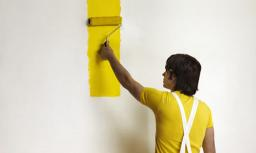Did you find an error or inaccuracy? Feel free to write us. Thank you!Tips to related online calculators
Tip: Our volume units converter will help you with the conversion of volume units.

#### You need to know the following knowledge to solve this word math problem:

We encourage you to watch this tutorial video on this math problem:

## Related math problems and questions:

• Painting a hutIt is necessary to paint the exterior walls of hut whose layout is a rectangle of 6.16 m x 8.78 m wall height is 2.85 meters. Cottage has five rectangular windows; three have dimensions of 1.15 m x 1.32 m and two 0,45 m x 0.96 m. How many m2 is necessary
• Jared's room paintingJared wants to paint his room. The room's dimensions are 12 feet by 15 feet, and the walls are 9 feet high. Two windows measure 6 feet by 5 feet each. There are two doors, whose dimensions are 30 inches by 6 feet each. If a gallon of paint covers approxim
• Room dimensions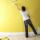Room dimensions are 5m and 3.5m. Room height is 2.85m. Paint the room (even with the ceiling). There will be 2 layers. Doors and windows have a total of 2.5 m2. One box of paint is enough for 6m2. How many boxes of paint are needed? How much do we pay if
• PaintingTo paint the pool with dimensions: 2 meters depth, 3m x 4m we bought paint to 50 meters square. How many "paint" will be a waste?
• Two sides paintThe door has the shape of a rectangle with dimensions of 260cm and 170cm. How many cans of paint will be needed to paint this door if one can of paint cover 2m2 of the area? We paint the doors on both sides.
• Markus painterMarkus used ¾ liter of paint to cover 10 ½ square meters of wall. How many liters of paint is needed to cover 12 ¼ square meters of wall?
• Mrs Holland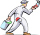Mrs Holland wants to paint her garage wall. The wall measures 6 m x 3 m Each can of paint covers 5m². Each can costs £7.50 How much will it cost Mrs Holland to paint her garage wall?
• In the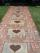In the garden, workers will pave a 1-meter-wide sidewalk with tiles around the block-shaped pool. The dimensions of the bottom of the pool are 8.5 meters and 6 meters. The height of the pool walls is 2 meters. How many m2 of pavement will be laid with til
• If one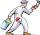If one liter of pants covers an area of 5 m2, how much paint is needed to cover: a) rectangular swimming pool With dimensions 4m x 3m x 2.5m (the Inside walls and the floor only) b) the Inside walls and floor of a cylindrical reservoir with a diameter of
• The wall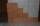The wall in the bathroom has dimensions of 2.8 m and 2.6 m. If we would like to cover this wall to a height of 1.6 m. How many square meters of tiles do we need to buy?
• Paint cansHow many paint cans do we need to paint the floor in two rooms with dimensions of 6.8m x 4.5m and 6m x3.8m? One can are for 6m².
• PaulaPaula is painting six doors that are all the same size. She used 2 liters of paint to cover 1 3/5 doors. How many liters of paint are needed for 1 door?
• Pool tilesThe pool is 25m long, 10m wide, and 160cm deep. How many m2 of tiles will be needed on the walls and the pool? How many tiles are needed when 1 tile has a square shape with a 20cm side? How much does it cost when 1m2 of tiles costs 258 Kc?
• How many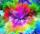How many cans of blue paint need to be bought if the interior of the garden pool, which is 5 m long, 3 m wide and 1 m deep, is to be painted? There is 1 kg of paint in each can. One can is enough for 8 m2 of area.
• Flower bed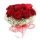The park has a rectangular flower bed with dimensions of 3.2m and 1.5m. How many rose bushes will we plant in the flower bed if 20 dm2 are needed for one bush?
• Sand pathHow many m3 of sand is needed to fill the 1.5m wide path around a rectangular flowerbed of 8m and 14m if the sand layer is 6cm high?
• PitThe pit has the shape of a truncated pyramid with a rectangular base and is 0.8 m deep. The pit's length and width are the top 3 × 1.5 m bottom 1 m × 0.5 m. To paint one square meter of the pit we use 0.6 l of green color. How many liters of paint are nee